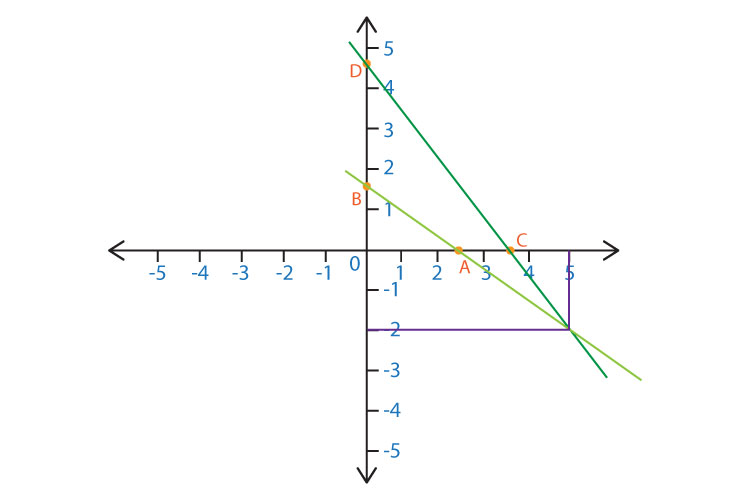# How to solve simultaneous equations with two unknowns?

The two main methods that you can solve simultaneous equations with two unknowns are:
Algebraically
○ Substitution Method
○ Elimination Method
Graphically

For Example we have the following set of linear simultaneous equations:

4x + 3y = 14 → Equation ①
5x + 7y = 11 → Equation ②

SUBSTITUTION METHOD
Let’s begin with solving this algebraically first. The first method I will be demonstrating is the substitution method

Step 1: Pick a variable
Let’s pick x. You can also pick y. There is no restriction, but in this case, I'm choosing x.

Step 2: Pick an equation
Let’s choose Equation ①
4x + 3y = 14

Step 3: Make x the subject in the selected equation
4x + 3y = 14
You begin with subtracting 3x from both sides.
4x + 3y - 3y = 14 - 3y
4x= 14 - 3y

Now you divide both sides by 4.
4x ÷ 4 = (14 - 3y) ÷ 4
x= (14 - 3y) ÷ 4 → Equation ③

Step 4: Substitute Equation ③ in Equation ②  in the place of x and solve the equation for y

5[(14 - 3y) ÷ 4] + 7y = 11    →You substitute x with equation ③
[(70 - 15y) ÷ 4] + 7y = 11    → You multiply 5 with 14 - 3y
70 - 15y + 4(7y) = 11(4)      → You multiply the entire equation by 4 to get rid of the fraction
70 - 15y + 28y = 44                 → Subtract 70 from both sides
28y - 15y = 44 -70       → Collect the like terms on each side
13y = ⁻ 26                    → Divide both sides by 13
y = ⁻ 2

Step 5: You substitute this value of y in Equation ③ to calculate the value of x

x = (14 - 3y) ÷ 4
= (14 - 3[-2]) ÷ 4
= ( 14 + 6) ÷ 4
= 20 ÷ 4
= 5

ELIMINATION METHOD

We’ll be using the same set of linear simultaneous equations for this method as well. In contrast to the previous method, in this one, the main focus is to eliminate one variable, so that equation just contains one unknown variable and not two.

4x + 3y = 14 → Equation ①
5x + 7y = 11 → Equation ②

Step 1: Pick a variable
I’ll be choosing variable y this time.

Step 2: Make sure that the coefficient of the chosen variable is equal in both equations
If you look at both the equations, in Equation ①, the coefficient of y is 3 and in Equation ② it is 7. Hence we need to make sure that they are the same. In order to achieve that, we will multiply Equation ① by 7 and Equation ② by 3.

7×(4x + 3y = 14)
3×(5x + 7y = 11)

28x + 21y = 98
15x + 21y = 33

Step 3: Subtract the two equations
Since both the 21y’s in the equations have a positive sign, we subtract the two equations to completely eliminate y from the equation. If the 21y’s had opposite signs, then we’d be adding the two equations.

28x + 21y = 98
-(15x + 21y = 33)

28x - 15x = 98 - 33
13x = 65
x= 65/ 13
x= 5

Step 4: Substitute this value of x in either of the equations to calculate the value of y

4x + 3y = 14
4(5) + 3y = 14
20 + 3y = 14
20- 20 + 3y = 14 - 20
3y = -6
y= -6/3
y= -2

As you can see, both methods give the same answers for x and y.

GRAPHICALLY
When it comes to solving equations graphically, it focuses on plotting the equations on a grid and the intersection of the two equations basically gives the value of x and y.

Step 1: Find the x- intercept and y-intercept of both the equations

4x + 3y = 14 → Equation ①

X- intercept is that point where the graph cuts the x-axis. For the x-intercept we put y = 0 in the equation.

4x + 3(0) = 14
4x = 14
x = 14/4
x = 3.5

Y-intercept is that point where your graph cuts the y-axis. For the y-intercept, we put x = 0 in the equation.

4(0) + 3y = 14
3y = 14
y = 14/3
y = 4.67

C( 3.5, 0) and D( 0, 4.67)

We repeat the same steps for equation 2

5x + 7y = 11 → Equation ②

For x-intercept:

5x + 7(0) = 11
5x = 11
x = 11/5
x = 2.5

For y-intercept:

5(0) + 7y = 11
7y = 11
y = 11/7
y = 1.57

A( 2.5, 0) and B( 0, 1.57)

Now that we have found the coordinates, we plot these on the axis and construct a graph for both the lines.The intersection of the two lines gives us the solution for these simultaneous equations, which is the same as the answers found through the previous methods, x=5 and y=-2.